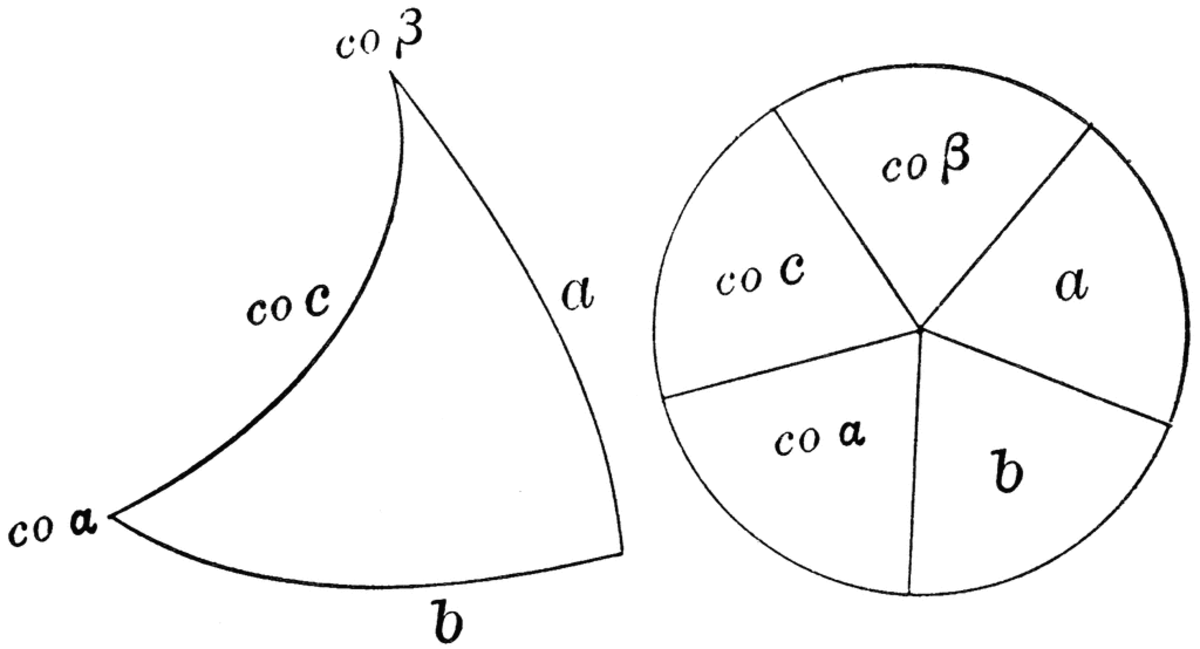# Right Spherical Triangles

Jinky is a 5th year Civil Engineering Student. She wants to share some tips on how to solve Math problems easily.Figure in the left is the Right Spherical Triangle ABC. Figure on the right is the Napier's Circle.

## Spherical Triangle

Spherical trigonometry is the branch of spherical geometry that deals with the relationships between trigonometric functions of the sides and angles of the spherical polygons defined by a number of intersecting great circles on the sphere.

A spherical triangle is a figure formed on the surface of a sphere by three great circular arcs intersecting pairwise in three vertices. The spherical triangle is the spherical analog of the planar triangle, and is sometimes called an Euler triangle (Harris and Stocker 1998). Let a spherical triangle have angles , , and (measured in radians at the vertices along the surface of the sphere) and let the sphere on which the spherical triangle sits have radius .A right spherical triangle, on the other hand, is a spherical triangle whose one of its angles measures 90°.

Spherical triangles are labeled with angles A, B and C, and respective sides a, b, and c opposite these angles. For right spherical triangles, it is customary to set C = 90°.

One way of solving for the missing sides and angles of a right spherical triangle is using Napier’s rules. Napier’s rules consist of two parts, and are used in conjunction with a figure called Napier’s circle as shown. Briefly stated,

## Rules

Rule 1: The SINe of a missing part is equal to the product of the TAngents of its ADjacent parts (SIN-TA-AD rule).

Rule 2: The SINe of a missing part is equal to the product of the COsine of its OPposite parts (SIN-CO-OP rule).

## Example

A spherical triangle ABC has an angle C = 90° and sides a = 50° and c = 80°.
1. Find angle B.
2. Find angle A.
3. Find side b.

## Solution

Since C = 90°, ABC is a right spherical triangle, and Napier’s rules will apply to the triangle. First, let us draw the Napier’s circle and highlight the given sides and angles. Remember the correct order: a, b, co-A, co-C, co-B.

1. Find angle B.
We are asked to find angle B, but we only have co-B. Notice that co-B is adjacent to co-c and a. The keyword here is “adjacent”. Hence, we use SIN-TA-AD rule.

sine of something = tangents of adjacents
sin(co-B) = tan(co-c) × tan(a)
sin(90° - B) = tan(90° - c) × tan(a) [Note: co-θ = 90° - θ.]
cos(B) = cot(c) × tan(a) [Note: We use cofunction identities in trigo.]
cos(B) = cot(80°) × tan(50°)
cos(B) = 0.2101
[B = 77°52’]

Now that we have found angle B, highlight this in the Napier’s circle as given.

Scroll to Continue

2. Find angle A
We are asked to find angle A, but we only have co-A. Notice that co-A is opposite a and co-B. The keyword here is “opposite”. Therefore, we use SIN-CO-OP rule.

sine of something = cosine of opposites
sin(co-A) = cos(a) × cos(co-B)
sin(90° - A) = cos(a) × cos(90° - B)
cos(A) = cos(a) × sin(B)
cos(A) = cos(50°) × sin(77°52’)
cos(A) = 0.6284
[A = 51°04’]

Now that we have found angle A, highlight this in the Napier’s circle as given.

3. Find side b [Please study the solution below carefully].
We are asked to find side b. Because cosines do not lead to ambiguous cases as compared to sines, we must try to put co-A, co-c or co-B in the sine part of our equation.

One way to do this is to note that co-c is opposite a and b. So, we use SIN-CO-OP rule.

sine of something = cosine of opposites
sin(co-c) = cos(a) × cos(b)
sin(90° - c) = cos(a) × cos(b)
cos(c) = cos(a) × cos(b)
cos(80°) = cos(50°) × cos(b)
cos(b) = cos(80°)/cos(50°)
cos(b) = 0.2701
[b = 74°20’]

Jinky Marie Manalo (author) from 126 San Joaquin Sto. Tomas Batangas on July 04, 2020:

Hi Spencer Skidmore, make it in a format of degrees and minutes not in decimal. Thanks!

Spencer Skidmore on July 04, 2020:

Double check your math on this.

B is 77.87

A is 50.29

For example. Calculator mishap?